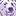# QlikView App Dev

Discussion Board for collaboration related to QlikView App Development.

Announcements
cancel
Showing results for
Did you mean:Creator

## Script load Error in expression: Nested aggregation not allowed

Hi all,

I am having some trouble replicating a (working set analysis formula) to load in script as I wish to export the results to a table

and keep getting Error in expression: Nested aggregation not allowed

the working variable set analysis syntax

SL_ON_HAND_QTY-

if(Sum({\$<TRANS_TYPE={''AAA'',''BBB''}>}ENTERED_QTY)>''0'',Sum({\$<TRANS_TYPE={''AAA'',''BBB''}>}ENTERED_QTY),''0'')

-

if(Sum({\$<TRANS_TYPE={''CCC''},STOCK_LOCATION={''2D44'',''2D55''}>}ENTERED_QTY)>=''0'',

Sum({\$<TRANS_TYPE={''CCC''},STOCK_LOCATION={''2D44'',''2D55''}>}ENTERED_QTY),''0'')

-

if(Sum({\$<TRANS_TYPE={''DDD''}>}ENTERED_QTY)>=''0'',Sum({\$<TRANS_TYPE={''DDD''}>}ENTERED_QTY),''0'')>0,

SL_ON_HAND_QTY-

if(Sum({\$<TRANS_TYPE={''AAA'',''BBB''}>}ENTERED_QTY)>''0'',Sum({\$<TRANS_TYPE={''AAA'',''BBB''}>}ENTERED_QTY),''0'')

-

if(Sum({\$<TRANS_TYPE={''CCC''},STOCK_LOCATION={''2D44'',''2D55''}>}ENTERED_QTY)>=''0'',

Sum({\$<TRANS_TYPE={''CCC''},STOCK_LOCATION={''2D44'',''2D55''}>}ENTERED_QTY),''0'')

-

if(Sum({\$<TRANS_TYPE={''DDD''}>}ENTERED_QTY)>=''0'',Sum({\$<TRANS_TYPE={''DDD''}>}ENTERED_QTY),''0''),0)

My attempt to replicate above .....load in script below  any help on correct way to handle this appreciated

Error in expression:

Nested aggregation not allowed

CaptureTotals:

Sum(SL_ON_HAND_QTY*STANDARD_COST) as TOTAL_OH_QTY,

SUM(SL_ON_HAND_QTY)-

SUM(if(MATCH(TRANS_TYPE,'AAA','BBB',ENTERED_QTY)>='0', Sum(MATCH(TRANS_TYPE,'AAA','BBB', ENTERED_QTY)))),'0'-

SUM(if(MATCH(TRANS_TYPE,'CCC' AND MATCH(STOCK_LOCATION,'2D44','2D55',ENTERED_QTY)>0,sum(MATCH(TRANS_TYPE,'CCC' AND MATCH(STOCK_LOCATION,'2D44','2D55'),ENTERED_QTY))),'0'-

SUM(if(MATCH(TRANS_TYPE,'DDD',ENTERED_QTY)>0,sum(MATCH(TRANS_TYPE,'DDD',ENTERED_QTY)))))),

SUM(SL_ON_HAND_QTY)-

SUM(IF(MATCH(TRANS_TYPE,'AAA','BBB',ENTERED_QTY)>='0', Sum(MATCH(TRANS_TYPE,'AAA','BBB', ENTERED_QTY)))),'0'-

SUM(IF(MATCH(TRANS_TYPE,'CCC' AND MATCH(STOCK_LOCATION,'2D44','2D55',ENTERED_QTY)>0,sum(MATCH(TRANS_TYPE,'CCC' AND MATCH(STOCK_LOCATION,'2D44','2D55'),ENTERED_QTY))),'0')-

SUM(IF(MATCH(TRANS_TYPE,'DDD',ENTERED_QTY)>0,sum(MATCH(TRANS_TYPE,'DDD',ENTERED_QTY)))))  AS SlowQtyScript

Resident FLAGS

where STOCK_LOCATION = '2D44'

thanks Peter

3 RepliesMVP

Mainly debug and highlight that line. Then we can better understand

Before develop something, think If placed (The Right information | To the right people | At the Right time | In the Right place | With the Right context)Creator
Author

Hi Anil,

that is exactly what I have done copy and pasted the debug screen above with what it returned

directly below where the  "Error in expression:"  is.

thanks PeterMVP

In fact, it is weird contract if we required in script. But, Try a luck use RangeSum() for your 2 Sum() expressions. I don't prefer to use this as script due to nested aggregation issue. Can this possible to use same in UI rather Script.

Before develop something, think If placed (The Right information | To the right people | At the Right time | In the Right place | With the Right context)